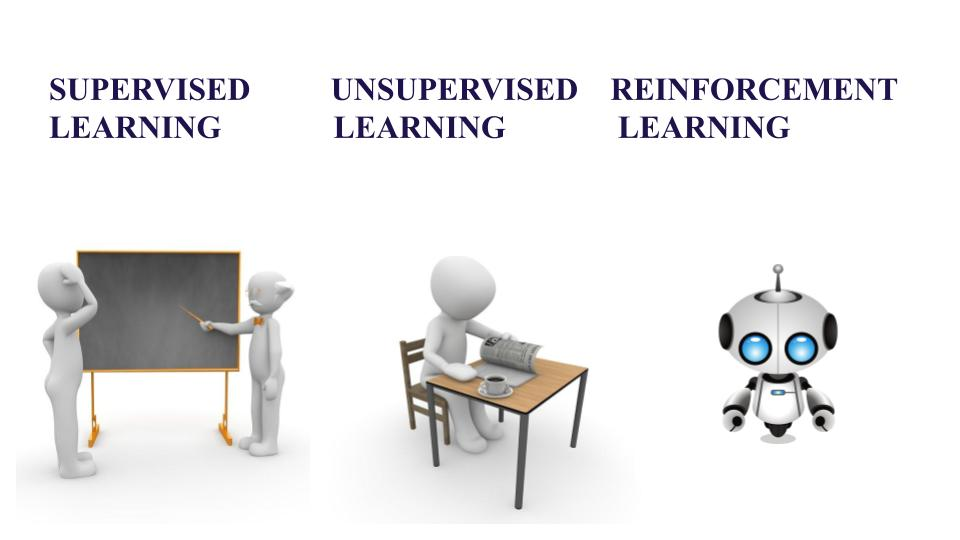The amount of data generated in the world today is very huge. This data is generated not only by humans but also by smartphones, computers and other devices. Based on the kind of data available and a motive present, certainly, a programmer will choose how to train an algorithm using a specific learning model.

Machine Learning is a part of Computer Science where the efficiency of a system improves itself by repeatedly performing the tasks by using data instead of explicitly programmed by programmers. Further let us understand the difference between three techniques of Machine Learning- Supervised, Unsupervised and Reinforcement Learning.

## Supervised Learning

Consider yourself as a student sitting in a classroom wherein your teacher is supervising you, “how you can solve the problem” or “whether you are doing correctly or not”. Likewise, in Supervised Learning input is provided as a labelled dataset, a model can learn from it to provide the result of the problem easily.

### Types of Problems

Supervised Learning deals with two types of problem- classification problems and regression problems.

#### Classification problems

This algorithm helps to predict a discrete value. It can be thought, the input data as a member of a particular class or group. For instance, taking up the photos of the fruit dataset, each photo has been labelled as a mango, an apple, etc. Here, the algorithm has to classify the new images into any of these categories. Examples:

• Naive Bayes Classifier
• Support Vector Machines
• Logistic Regression

#### Regression problems

These problems are used for continuous data. For example, predicting the price of a piece of land in a city, given the area, location, number of rooms, etc. And then the input is sent to the machine for calculating the price of the land according to previous examples. Examples-

• Linear Regression
• Nonlinear Regression
• Bayesian Linear Regression

## Unsupervised Learning

This learning algorithm is completely opposite to Supervised Learning. In short, there is no complete and clean labelled dataset in unsupervised learning. Unsupervised learning is self-organized learning. Its main aim is to explore the underlying patterns and predicts the output.  Here we basically provide the machine with data and ask to look for hidden features and cluster the data in a way that makes sense. Example

• K – Means clustering
• Neural Networks
• Principal Component Analysis

## Reinforcement Learning

It is neither based on supervised learning nor unsupervised learning. Moreover, here the algorithms learn to react to an environment on their own. It is rapidly growing and moreover producing a variety of learning algorithms. These algorithms are useful in the field of Robotics, Gaming etc.

For a learning agent, there is always a start state and an end state. However, to reach the end state, there might be a different path. In Reinforcement Learning Problem an agent tries to manipulate the environment. The agent travels from one state to another. The agent gets the reward(appreciation) on success but will not receive any reward or appreciation on failure. In this way, the agent learns from the environment.

### Key Differences Between Supervised vs Unsupervised Learning vs Reinforcement Learning

1. Supervised Learning deals with two main tasks Regression and Classification. Unsupervised Learning deals with clustering and associative rule mining problems. Whereas Reinforcement Learning deals with exploitation or exploration, Markov’s decision processes, Policy Learning, Deep Learning and value learning.
2. Supervised Learning works with the labelled data and here the output data patterns are known to the system. But, the unsupervised learning deals with unlabeled data where the output is based on the collection of perceptions. Whereas in Reinforcement Learning Markov’s Decision process- the agent interacts with the environment in discrete steps.
3. The name itself says, Supervised Learning is highly supervised. And Unsupervised Learning is not supervised. As against, Reinforcement Learning is less supervised which depends on the agent in determining the output.
4. The input data in Supervised Learning in labelled data. Whereas, in Unsupervised Learning the data is unlabelled. The data is not predefined in Reinforcement Learning.
5. Supervised Learning predicts based on a class type. Unsupervised Learning discovers underlying patterns. And in Reinforcement Learning, the learning agent works as a reward and action system.
6. Supervised learning maps labelled data to known output. Whereas, Unsupervised Learning explore patterns and predict the output. Reinforcement Learning follows a trial and error method.
7. To sum up, in Supervised Learning, the goal is to generate formula based on input and output values. In Unsupervised Learning, we find an association between input values and group them. In Reinforcement Learning an agent learn through delayed feedback by interacting with the environment.